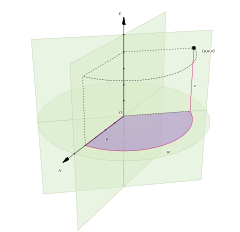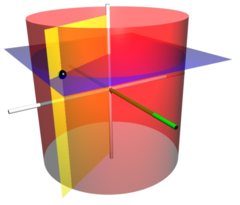# Cylindrical coordinate system

﻿
Cylindrical coordinate systemA cylindrical coordinate system with origin O, polar axis A, and longitudinal axis L. The dot is the point with radial distance ρ = 4, angular coordinate φ = 130°, and height z = 4.

A cylindrical coordinate system is a three-dimensional coordinate system that specifies point positions by the distance from a chosen reference axis, the direction from the axis relative to a chosen reference direction, and the distance from a chosen reference plane perpendicular to the axis. The latter distance is given as a positive or negative number depending on which side of the reference plane faces the point.

The origin of the system is the point where all three coordinates can be given as zero. This is the intersection between the reference plane and the axis.

The axis is variously called the cylindrical or longitudinal axis, to differentiate it from the polar axis, which is the ray that lies in the reference plane, starting at the origin and pointing in the reference direction.

The distance from the axis may be called the radial distance or radius, while the angular coordinate is sometimes referred to as the angular position or as the azimuth. The radius and the azimuth are together called the polar coordinates, as they correspond to a two-dimensional polar coordinate system in the plane through the point, parallel to the reference plane. The third coordinate may be called the height or altitude (if the reference plane is considered horizontal), longitudinal position, or axial position.

Cylindrical coordinates are useful in connection with objects and phenomena that have some rotational symmetry about the longitudinal axis, such as water flow in a straight pipe with round cross-section, heat distribution in a metal cylinder, and so on.

## Definition

The three coordinates (ρ, φ, z) of a point P are defined as:

• The radial distance ρ is the Euclidean distance from the z axis to the point P.
• The azimuth φ is the angle between the reference direction on the chosen plane and the line from the origin to the projection of P on the plane.
• The height z is the signed distance from the chosen plane to the point P.

### Unique cylindrical coordinates

As in polar coordinates, the same point with cylindrical coordinates (ρ, φ, z) has infinitely many equivalent coordinates, namely (ρ, φ ± n×360°, z) and (−ρ, φ ± (2n + 1)×180°, z), where n is any integer. Moreover, if the radius ρ is zero, the azimuth is arbitrary.

In situations where one needs a unique set of coordinates for each point, one may restrict the radius to be non-negative (ρ ≥ 0) and the azimuth φ to lie in a specific interval spanning 360°, such as (−180°,+180°] or [0,360°).

### Conventions

The notation for cylindrical coordinates is not uniform. The ISO standard 31-11 recommends (ρ, φ, z), where ρ is the radial coordinate, φ the azimuth, and z the height. However, the radius is also often denoted r, the azimuth by θ or t, and the third coordinate by h or (if the cylindrical axis is considered horizontal) x, or any context-specific letter.The coordinate surfaces of the cylindrical coordinates (ρ, φ, z). The red cylinder shows the points with ρ=2, the blue plane shows the points with z=1, and the yellow half-plane shows the points with φ=−60°. The z-axis is vertical and the x-axis is highlighted in green. The three surfaces intersect at the point P with those coordinates (shown as a black sphere); the Cartesian coordinates of P are roughly (1.0, −1.732, 1.0).Cylindrical Coordinate Surfaces. The three orthogonal components, ρ (green), φ (red), and z (blue), each increasing at a constant rate. The point is at the intersection between the three colored surfaces.

In concrete situations, and in many mathematical illustrations, a positive angular coordinate is measured counterclockwise as seen from any point with positive height.

## Coordinate system conversions

The cylindrical coordinate system is one of many three-dimensional coordinate systems. The following formulae may be used to convert between them.

### Cartesian coordinates

For the conversion between cylindrical and Cartesian coordinate systems, it is convenient to assume that the reference plane of the former is the Cartesian xy plane (with equation z = 0) , and the cylindrical axis is the Cartesian z axis. Then the z coordinate is the same in both systems, and the correspondence between cylindrical (ρ,φ) and Cartesian (x,y) are the same as for polar coordinates, namely

x = ρcos φ
y = ρsin φ

in one direction, and$\rho = \sqrt{x^{2}+y^{2}}$$\varphi = \begin{cases} 0 & \mbox{if } x = 0 \mbox{ and } y = 0\\ \arcsin(\frac{y}{\rho}) & \mbox{if } x \geq 0 \\ -\arcsin(\frac{y}{\rho}) + \pi & \mbox{if } x < 0\\ \end{cases}$

in the other. The arcsin function is the inverse of the sine function, and is assumed to return an angle in the range [−π/2,+π/2] = [−90°,+90°]. These formulas yield an azimuth φ in the range [−90°,+270°]. For other formulas, see the polar coordinate article.

Many modern programming languages provide a function that will compute the correct azimuth φ, in the range (−π, π], given x and y, without the need to perform a case analysis as above. For example, this function is called by atan2(y,x) in the C programming language, and atan(y,x) in Common Lisp.

### Spherical coordinates

Spherical coordinates (radius r, elevation or inclination θ, azimuth φ), may be converted into cylindrical coordinates by:

 θ is elevation: θ is inclination:$\rho = r \cos \theta \,$$\rho = r \sin \theta \,$$\varphi = \varphi \,$$\varphi = \varphi \,$$z = r \sin \theta \,$$z = r \cos \theta \,$

Cylindrical coordinates may be converted into spherical coordinates by:

 θ is elevation: θ is inclination:$r=\sqrt{\rho^2+z^2}$$r=\sqrt{\rho^2+z^2}$${\theta}=\operatorname{arcsin}(z/r)$${\theta}=\operatorname{arccos}(z/r)$${\varphi}=\varphi \quad$${\varphi}=\varphi \quad$

## Line and volume elements

See multiple integral for details of volume integration in cylindrical coordinates, and Del in cylindrical and spherical coordinates for vector calculus formulae.

In many problems involving cylindrical polar coordinates, it is useful to know the line and volume elements; these are used in integration to solve problems involving paths and volumes.

The line element is$\mathrm d\mathbf{r} = \mathrm d\rho\,\boldsymbol{\hat \rho} + \rho\,\mathrm d\varphi\,\boldsymbol{\hat\varphi} + \mathrm dz\,\mathbf{\hat z}.$

The volume element is$\mathrm{d}V = \rho\,\mathrm{d}\rho\,\mathrm{d}\varphi\,\mathrm{d}z.$

The surface element in a surface of constant radius ρ (a vertical cylinder) is$\mathrm{d}S_\rho= \rho\,\mathrm{d}\varphi\,\mathrm{d}z.$

The surface element in a surface of constant azimuth φ (a vertical half-plane) is$\mathrm{d}S_\varphi= \mathrm{d}\rho\,\mathrm{d}z.$

The surface element in a surface of constant height z (a horizontal plane) is$\mathrm{d}S_z= \rho\,\mathrm{d}\rho\,\mathrm{d}\varphi.$

The del operator in this system is written as$\nabla = \boldsymbol{\hat \rho}\frac{\partial}{\partial \rho} + \boldsymbol{\hat \varphi}\frac{1}{\rho}\frac{\partial}{\partial \varphi} + \mathbf{\hat z}\frac{\partial}{\partial z},$

and the Laplace operator$\nabla^2$ is defined by$\nabla^2 f = {1 \over \rho} {\partial \over \partial \rho} \left( \rho {\partial f \over \partial \rho} \right) + {1 \over \rho^2} {\partial^2 f \over \partial \varphi^2} + {\partial^2 f \over \partial z^2 }.$

## Cylindrical harmonics

The solutions to the Laplace equation in a system with cylindrical symmetry are called cylindrical harmonics.

Wikimedia Foundation. 2010.

### Look at other dictionaries:

• Coordinate system — For geographical coordinates on Wikipedia, see Wikipedia:WikiProject Geographical coordinates. In geometry, a coordinate system is a system which uses one or more numbers, or coordinates, to uniquely determine the position of a point or other… …   Wikipedia

• cylindrical coordinate — noun Date: circa 1934 any of the coordinates in space obtained by constructing in a plane a polar coordinate system and on a line perpendicular to the plane a linear coordinate system …   New Collegiate Dictionary

• coordinate system — Math. any method that uses numbers to represent a point, line, or the like. * * * Arrangement of reference lines or curves used to identify the location of points in space. In two dimensions, the most common system is the Cartesian (after René… …   Universalium

• cylindrical coordinate — noun mathematics : any of the coordinates in space obtained by constructing in a plane a polar coordinate system and on a line perpendicular to the plane at the pole a linear coordinate system …   Useful english dictionary

• Polar coordinate system — Points in the polar coordinate system with pole O and polar axis L. In green, the point with radial coordinate 3 and angular coordinate 60 …   Wikipedia

• Spherical coordinate system — In mathematics, the spherical coordinate system is a coordinate system for representing geometric figures in three dimensions using three coordinates: the radial distance of a point from a fixed origin, the zenith angle from the positive z axis… …   Wikipedia

• Cartesian coordinate system — Illustration of a Cartesian coordinate plane. Four points are marked and labeled with their coordinates: (2, 3) in green, (−3, 1) in red, (−1.5, −2.5) in blue, and the origin (0, 0) in purple. A Cartesian coordinate system specifies each point… …   Wikipedia

• Coordinate-measuring machine — A coordinate measuring machine (CMM) is a device for measuring the physical geometrical characteristics of an object. This machine may be manually controlled by an operator or it may be computer controlled. Measurements are defined by a probe… …   Wikipedia

• Vector fields in cylindrical and spherical coordinates — Cylindrical coordinate system = Vector fields Vectors are defined in cylindrical coordinates by (ρ,φ,z), where * ρ is the length of the vector projected onto the X Y plane, * φ is the angle of the projected vector with the positive X axis (0 ≤ φ… …   Wikipedia

• z-coordinate — ˈ ̷ ̷ ̷ ̷ˈ ̷ ̷( ̷ ̷) ̷ ̷ noun 1. : one of the three coordinates in a three dimensional rectangular coordinate system 2. : the third coordinate in a cylindrical coordinate system …   Useful english dictionary